Next: 6. Visuomotor model for Up: 5. Kernel PCA for Previous: 5.3.2.5 Kinematic arm model

# 5.4 Discussion

A new pattern-association model was introduced. A potential field based on the distribution of training patterns was constructed. In an infinite-dimensional feature space--into which the patterns are virtually mapped--the potential is the squared distanced to a subspace spanned by the principal components. The corresponding field is called cylindrical. Instead of computing in the feature space, the algorithm uses kernel PCA in the original space.

The potential field gained from two-dimensional synthetic distributions resembled accurately the shape of these distributions. Here, the cylindrical potential field in feature space could describe a distribution better than a spherical potential field. The reason is that the cylindrical field accounts for the non-uniform variance in feature space (for ring-line-square, 40 out of 850 principal components described 94.5% of the variance). This comparison also shows that the cylindrical potential field is better than the classical Parzen window density estimator (see section 5.2.1). Given the promising performance on this task, the model could also be applied to novelty detection.

The algorithm needs only two parameters: the number of principal components q and the width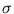of the Gaussian kernel. The first gives a control on the complexity of the approximation of the data distribution. In the absence of noise, increasing q increases the quality of the potential field. The number q can be estimated with the help of the amount of variance explained by the principal components; for example, 99% explained variance implies that is it useless to extract more components.

The widthshould be adjusted to about 10% to 50% of the square-root of the total variance of the distribution (the sum over all eigenvalues of the covariance matrix). Small changes seem to have a minor influence on the quality (table 5.2). While for higherthe principal components explain more of the distribution's variance, the wider Gaussian functions occlude more details.

In recall, the conjugate gradient method was used to minimize the potential field along a subspace whose offset from zero is set by the input to the network. It was demonstrated that the recall algorithm works well on a synthetic distribution, despite the noise in the training set. In contrast, in the models using all training patterns as attractors (Bachmann et al., 1987; Dembo and Zeitouni, 1988), noise points are also attractors, and therefore lead to undesired completions.

The presented relaxation model was further tested on a kinematic arm model. It could cope with the one-to-many mapping (a feed-forward network fails on this task, see section 4.5). The error on the forward mapping was higher (about double) than on the inverse mapping. The reason is possibly the same as discussed in section 4.6.

On the kinematic arm task, the local PCA mixture model did better then the kernel PCA model. The reason might be the multivariate shape of the ellipsoids. In contrast, the kernel PCA model is composed of univariate Gaussians in the original space (5.6). In the univariate case, many Gaussians might be needed to describe a multivariate structure, which can be described by a single ellipsoid (figure 3.1). The composition of univariate units also results in the sinus-shape-like approximation of a tilted line (figure 5.6, right).

On the kinematic arm data, the mixture model NGPCA was at a limit. With fewer training patterns as in section 4.5, also the number of units needed to be smaller. However, adding more patterns does not necessarily require a longer computation time. In section 4.5, the number of training steps was the same ( tmax = 400 000); thus, also the computation times were equal. In contrast, for kernel PCA, increasing the number of patterns n comes at a high cost (the kernel matrix is n×n).

Kernel PCA is computationally demanding. On an Athlon XP 2200+ with 1 GB RAM with a compiled C++ code of the algorithm, the computation of the kernel matrix and the 15 principal components for the sine-wave distribution took about 14 sec. The computation of the reduced set with m = 85 took 101 sec, and using the reduced set, the mean recall time was 0.058 sec. In comparison, on the same computer with the same distribution, the mixture model--which was composed of 10 units with two principal components each--had a training time of about 3.7 sec, and a mean recall time of 0.14 msec (about 400 times faster).

The cylindrical potential differs from the square error in the denoising application of kernel PCA (Mika et al., 1999). In denoising of a pattern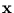, its mapping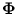() is projected onto the subspace spanned by the principal components in feature space--the same subspace we use for our potential. The denoised pattern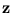is obtained by minimizing the squared distance between() and the projection of(). In contrast, our potential is the squared distance between() and its own projection onto the subspace. This difference is illustrated in figure 5.8.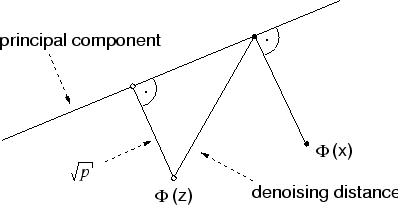Kernel PCA requires fewer parameters than a mixture model, but the pattern association based on kernel PCA did worse than the abstract RNN based on a mixture of local PCA. In the next chapter, both methods are applied to visually guided grasping.Next: 6. Visuomotor model for Up: 5. Kernel PCA for Previous: 5.3.2.5 Kinematic arm model
Heiko Hoffmann
2005-03-22